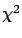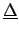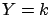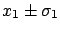Next: Offset uncertainty Up: Use and misuse of Previous: Use and misuse of   Contents

### Best estimate of the true value from two correlated values.

Once the covariance matrix is built one uses it in afit to get the parameters of a function. The quantity to be minimized is, defined as(6.47)

whereis the vector of the differences between the theoretical and the experimental values. Let us consider the simple case in which two results of the same physical quantity are available, and the individual and the common standard uncertainty are known. The best estimate of the true value of the physical quantity is then obtained by fitting the constantthrough the data points. In this simple case theminimization can be performed easily. We will consider the two cases of offset and normalization uncertainty. As before, we assume that the detector is well calibrated, i.e. the most probable value of the calibration constant is, respectively for the two cases, 0 and 1, and henceNext: Offset uncertainty Up: Use and misuse of Previous: Use and misuse of   Contents
Giulio D'Agostini 2003-05-15